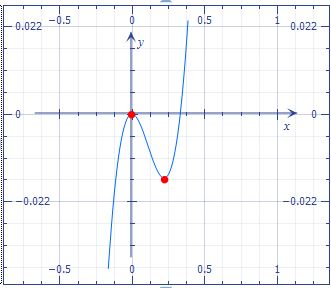# What is the maximum number of relative extrema contained in the graph of this function y=3x^3-x^2.

## Question:

What is the maximum number of relative extrema contained in the graph of this function {eq}y=3x^3-x^2 {/eq}.

## Relative Extreme:

The relative extremes (Maximum and Minimum) of a function are critical points at which the function reaches its maximum or minimum increase or decrease.

We have the function:

{eq}y=3x^3-x^2 \\ {/eq}

Graph the functionRelative maximum at: {eq}(0,0) \\ {/eq}

Relative minimum at: {eq}(\frac{2}{9}, -\frac{4}{243}) \\ {/eq}